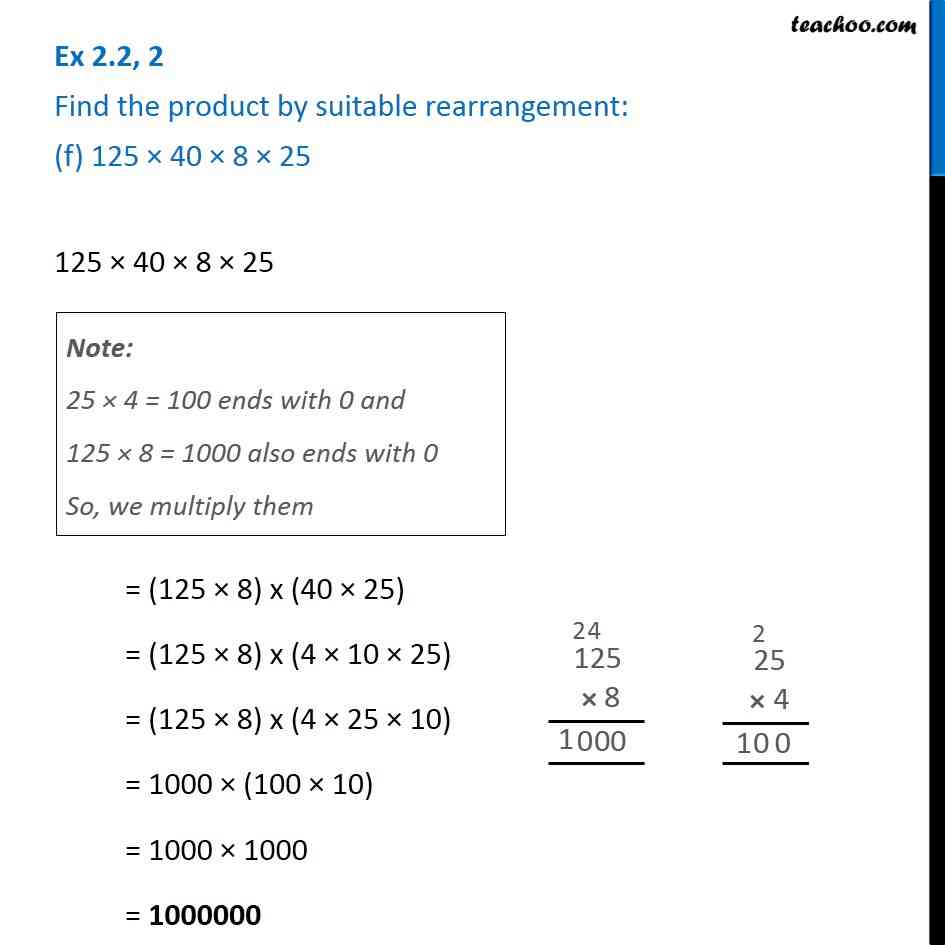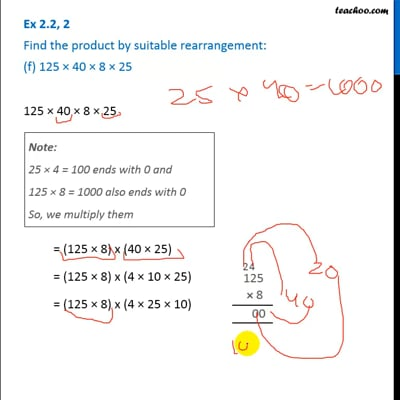Ex 2.2

Chapter 2 Class 6 Whole Numbers
Serial order wiseThis video is only available for Teachoo black users

Introducing your new favourite teacher - Teachoo Black, at only ₹83 per month

### Transcript

Ex 2.2, 2 Find the product by suitable rearrangement: (f) 125 × 40 × 8 × 25 125 × 40 × 8 × 25 = (125 × 8) x (40 × 25) = (125 × 8) x (4 × 10 × 25) = (125 × 8) x (4 × 25 × 10) = 1000 × (100 × 10) = 1000 × 1000 = 1000000 Note: 25 × 4 = 100 ends with 0 and 125 × 8 = 1000 also ends with 0 So, we multiply them